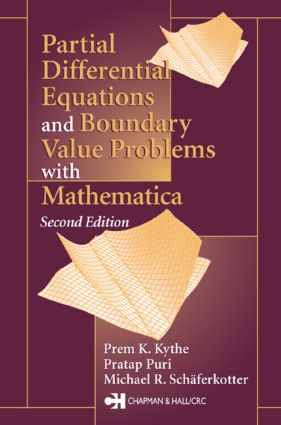Partial Differential Equations and Mathematica

2nd Edition

Chapman and Hall/CRC

440 pages | 74 B/W Illus.

Purchasing Options:\$ = USD
Hardback: 9781584883142
pub: 2002-11-12
SAVE ~\$27.00
\$135.00
\$108.00
x
eBook (VitalSource) : 9781315273105
pub: 2018-10-03
from \$67.50

FREE Standard Shipping!

Description

Early training in the elementary techniques of partial differential equations is invaluable to students in engineering and the sciences as well as mathematics. However, to be effective, an undergraduate introduction must be carefully designed to be challenging, yet still reasonable in its demands. Judging from the first edition's popularity, instructors and students agree that despite the subject's complexity, it can be made fairly easy to understand.

Revised and updated to reflect the latest version of Mathematica, Partial Differential Equations and Boundary Value Problems with Mathematica, Second Edition meets the needs of mathematics, science, and engineering students even better. While retaining systematic coverage of theory and applications, the authors have made extensive changes that improve the text's accessibility, thoroughness, and practicality.

New in this edition:

• Upgraded and expanded Mathematica sections that include more exercises

• An entire chapter on boundary value problems

• More on inverse operators, Legendre functions, and Bessel functions

• Simplified treatment of Green's functions that make it more accessible to undergraduates

• A section on the numerical computation of Green's functions

• Mathemcatica codes for solving most of the problems discussed

• Boundary value problems from continuum mechanics, particularly on boundary layers and fluctuating flows

• Wave propagation and dispersion

With its emphasis firmly on solution methods, this book is ideal for any mathematics curricula. It succeeds not only in preparing readers to meet the challenge of PDEs, but also in imparting the inherent beauty and applicability of the subject.

INTRODUCTION TO MATHEMATICA

Introduction

Conventions

Getting Started

File Manipulation

Differential Equations

To the Instructor

To the Student

MathSource

INTRODUCTION

Notation and Definitions

Initial and Boundary Conditions

Classification of Second Order Equations

Some Known Equations

Superposition Principle

METHOD OF CHARACTERISTICS

First Order Equations

Linear Equations with Constant Coefficients

Linear Equations with Variable Coefficients

First Order Quasi-Linear Equations

First Order Nonlinear Equations

Geometrical Considerations

Some Theorems on Characteristics

Second Order Equations

Linear and Quasi-linear Equations

LINEAR EQUATIONS WITH CONSTANT COEFFICIENTS

Inverse Operators

Homogeneous Equations

Nonhomogeneous Equations

ORTHOGONAL EXPANSIONS

Orthogonality

Orthogonal Polynomials

Series of Orthogonal Functions

Trigonometric Fourier Series

Eigenfunction Expansions

Bessel Functions

SEPARATION OF VARIABLES

Introduction

Hyperbolic Equations

Parabolic Equations

Elliptic Equations

Cylindrical Coordinates

Spherical Coordinates

Nonhomogeneous Problems

INTEGRAL TRANSFORMS

Laplace Transforms

Notation

Basic Laplace Transforms

Inversion Theorem

Fourier Transforms

Fourier Integral Theorems

Properties of Fourier Transforms

Fourier Sine and Cosine Transforms

Finite Fourier Transforms

GREEN'S FUNCTIONS

Generalized Functions

Green's Functions

Elliptic Equations

Parabolic Equations

Hyperbolic Equations

Applications of Green's Functions

Computation of Green's Functions

BOUNDARY VALUE PROBLEMS

Initial and Boundary Conditions

Implicit Conditions

Periodic Conditions

Wave Propagation and Dispersion

Boundary Layer Flows

Miscellaneous Problems

WEIGHTED RESIDUAL METHODS

Line Integrals

Variational Notation

Multiple Integrals

Weak Variational Formulation

Rayleigh-Ritz Method

Choice of Test Functions

Transient Problems

Other Methods

PERTURBATION METHODS

Perturbation Problem

Taylor Series Expansions

Successive Approximations

Boundary Perturbations

Fluctuating Flows

FINITE DIFFERENCE METHODS

Finite Difference Schemes

First Order Equations

Second Order Equations

Appendix A: Green's Identities

Appendix B: Orthogonal Polynomials

Appendix C: Tables of Transform Pairs

Appendix D: Glossary of Mathematica Functions

Appendix E: Mathematica Packages and Notebooks

Bibliography

Index

Each chapter also contains sections of Mathematica Projects and Exercises